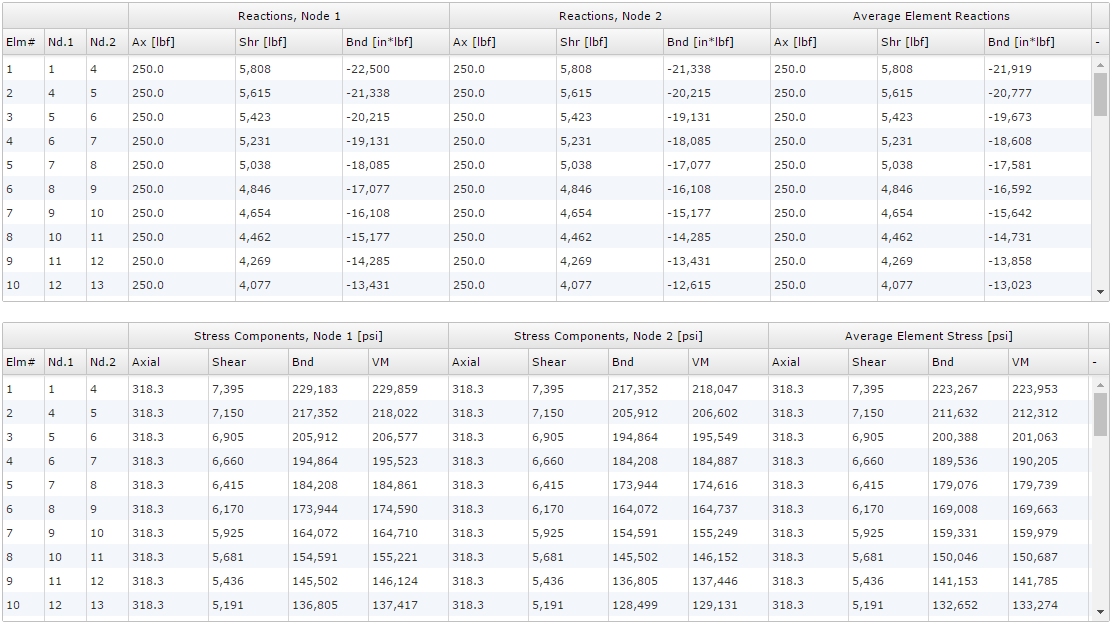﻿ Beam Analysis - Instructions | MechaniCalc

## Reference and Validation

Reference: A general description of the theory and the methodology used can be found here.

Validation: This tool has been validated against the known solutions to numerous example problems. Documentation of the validation can be found here.

## Inputs

Several categories of input are required, each of which corresponds to a tab on the Inputs page:

• Structure (defining the geometry and material)
• Forces
• Distributed Forces
• Constraints

An example of a beam model with fully defined structure, forces, and constraints is shown below: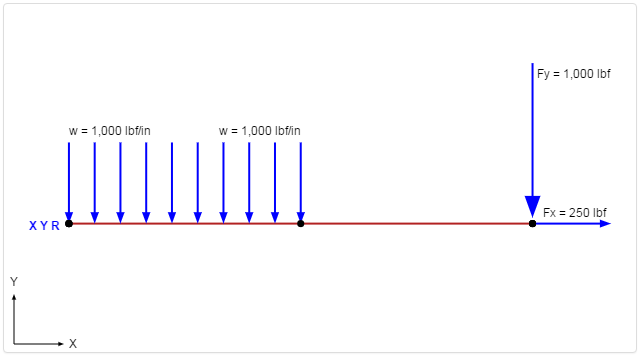### Structure

In the Structure tab, you define the geometry (length and cross section) and the material of the beam. In the example below, the beam is specified as 10 inches long with a circular 1 inch diameter cross section. The material is specified as Aluminum 6061, T6.

It should be noted that the materials and cross sections are managed on separate sections of the site. If the desired materials and cross sections do not exist in the drop-down lists, they can be added in those sections. The blue "New" buttons next to the material and cross section drop-down lists provide links to the appropriate sections.### Forces

The Forces tab allows you to apply concentrated (point) forces and moments to the beam. A force is specified by a location as well as values for applied FX (axial force), FY (transverse force), and M (moment). The location is specified with respect to the left side of the beam.

In the example below, an applied force is specified with an FX component of 250 lbf and an FY component of 1000 lbf (highlighted in red). No moment is specified.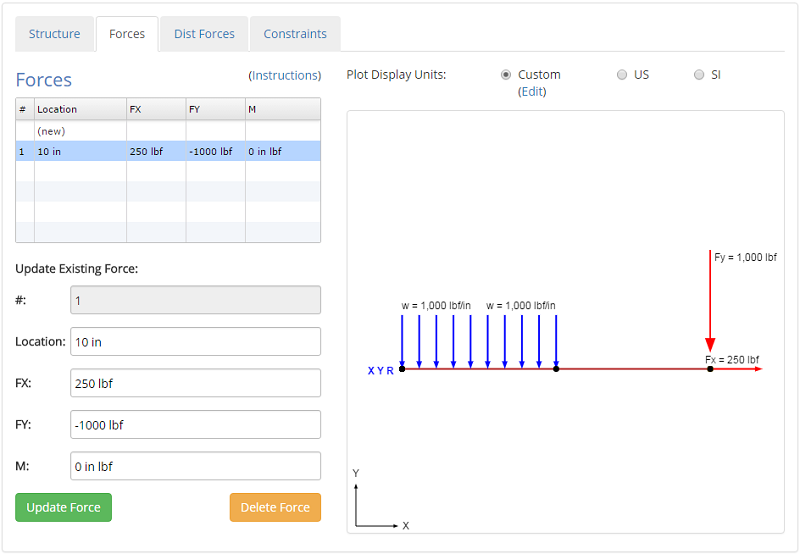### Distributed Forces

The Distributed Forces tab allows you to apply distributed forces to the beam. A distributed force is specified with a location and a force value at the start and end points. The locations are specified with respect to the left side of the beam. The distributed force values must have units of [force/length] (e.g. "lbf/in", "N/m", etc.).

In the example below, an uniform distributed force of 1000 lbf/in is specified starting at the left side of the beam and ending 5 inches along the length of the beam (highlighted in red).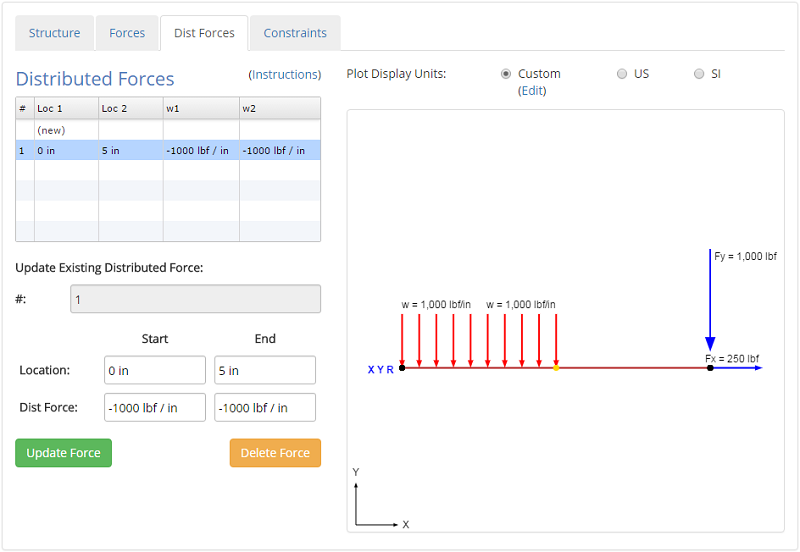### Constraints

The Constraints tab allows you to apply constraints to the beam. A constraint is specified with a location as well as fixed/free conditions for translation in X (TX), translation in Y (TY), and rotation (R). Each applied constraint is indicated in the model plot via text with the fixed degrees of freedom specified.

Common boundary conditions can be applied by specifying different combinations of constraints, as seen in the table below. The text that would be displayed on the model plot for each of these constraints is also provided in the table for reference.

Boundary ConditionTXTYRDisplay Text
FixedFixedFixedFixedX Y R
PinnedFixedFixedFreeX Y
Guided in XFreeFixedFixedY R
Guided in YFixedFreeFixedX R
Roller in XFreeFixedFreeY
Roller in YFixedFreeFreeX

In the example below, a fixed constraint is specified at the left side of the beam. The constraint is highlighted in red.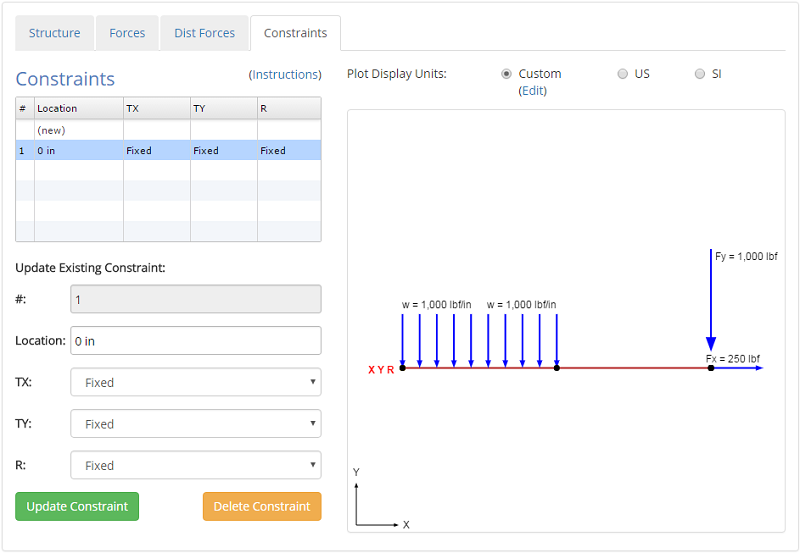## Viewing Results

There are a number of different results that are given as an output. These results include:

• Results Overview
• Shear-Moment Diagram
• Stress Plots
• Deflection Plots
• Result Tables

### Results Overview

In the Results Overview section, the Free Body Diagram (FBD) is given that shows the applied forces and the reaction forces on the beam. The deformed mesh is also given that shows the node displacements on an exaggerated scale. The scale for the deformed mesh is automatically chosen for optimal viewing.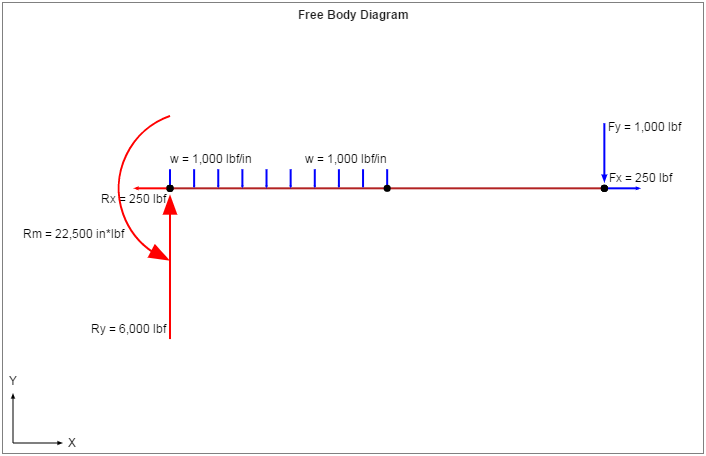### Shear-Moment Diagrams

The shear and moment diagrams for the current example are shown below. The standard sign conventions for shear-moment diagrams are followed:

• Shear: Positive shear causes clockwise rotation of the beam, negative shear causes counter-clockwise rotation.
• Moment: Positive moment will compress the top of the beam and elongate the bottom of the beam (i.e. make the beam 'smile').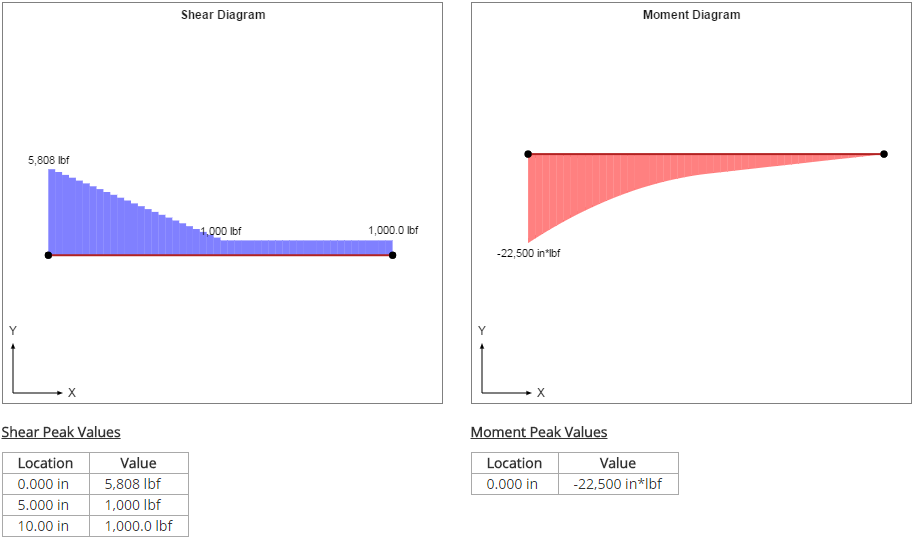### Stress Plots

The stress plots for the current example are shown below.The stresses are calculated based on the equations in the following table:

Axial Stress Shear Stress Bending Stress Von Mises Stress
$$\sigma_{ax} = {F_{ax} \over A}$$ $$\tau_{sh} = {F_{sh} \over A}$$ $$\sigma_{b} = {Mc \over I}$$ $$\sigma_{vm} = \sqrt{ (\sigma_{ax} + \sigma_{b})^2 + 3\tau_{sh}^2 }$$

### Deflection Plots

The deflection plots for the current example are shown below. The sign convention for deflections is:

• X: Positive to right, negative to left
• Y: Positive up, negative down
• Rotation: Right hand rule (positive counterclockwise, negative clockwise)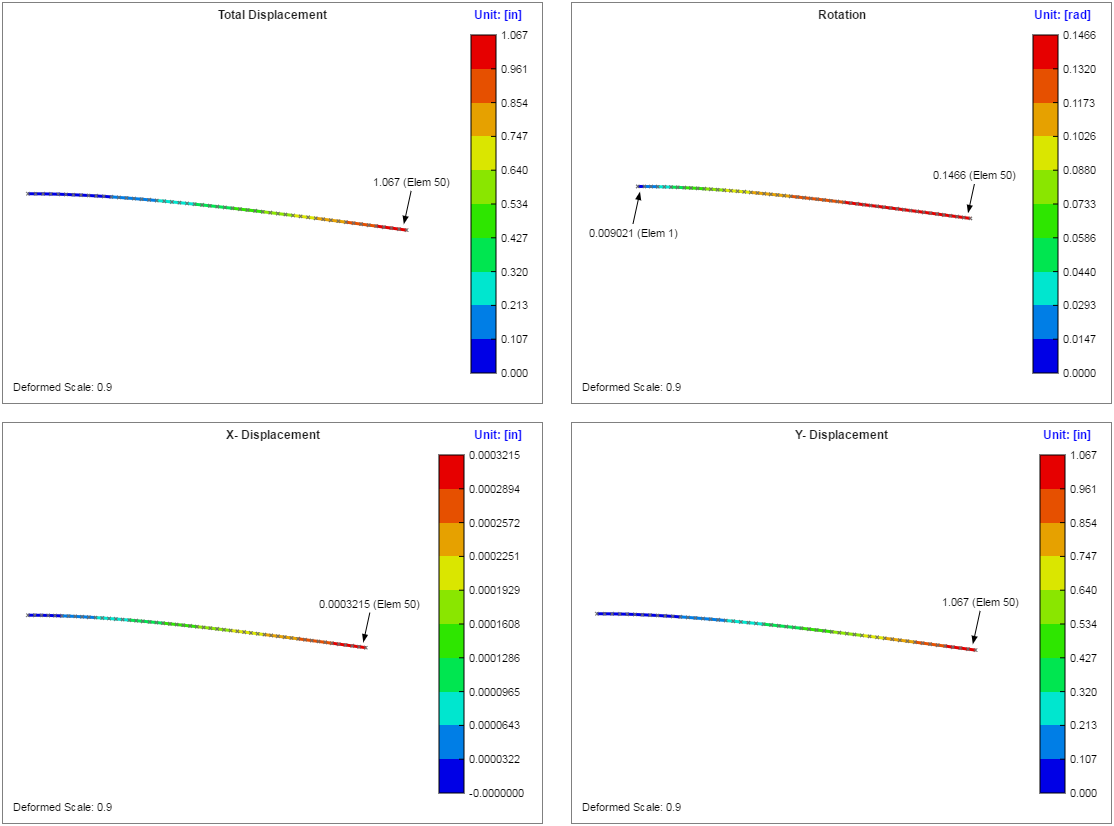### Result Tables

The beam analysis is solved as a finite element model. The plot below shows the mesh with the element numbers labeled: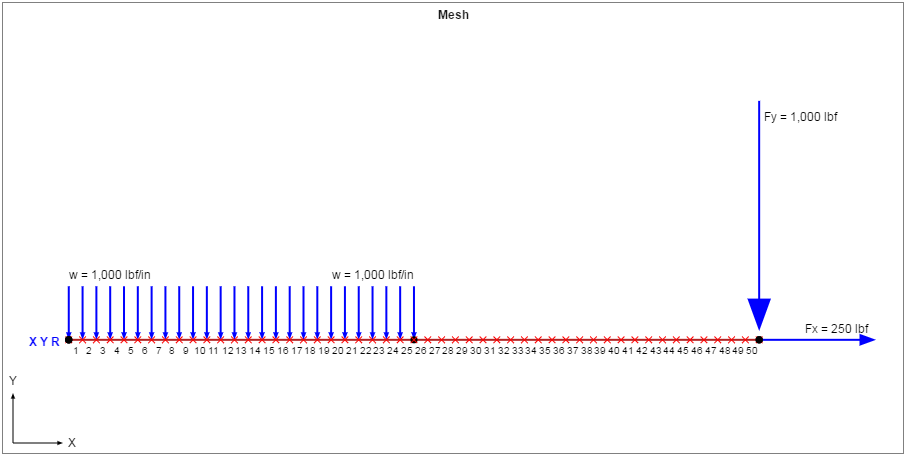The nodal result table for this example is shown below. This table shows the node numbers along with any associated point numbers (a node is created for every point in the model). The applied forces and constraints are shown on each node, where applicable, as well as the displacements and external reactions on each node. Note that external reactions will only be non-zero where constraints are applied.The elemental result tables for this example are shown below. Every element consists of two nodes; these nodes are referred to as "Node 1" and "Node 2" in the result tables. For example, in the first row of the table below it can be seen that Element 1 contains Node 1 and Node 4 in the mesh. For Element 1, "Node 1" refers to Node 1 and "Node 2" refers to Node 4.

In the first of the elemental result tables, the axial force, shear force, and bending moments are given for each node of each element. The average elemental forces are also given, which are simply the average of the nodal forces.

In the second of the elemental result tables, the axial, shear, bending, and von Mises stresses are given for each node of each element. The average elemental stresses are also given, which are simply the average of the nodal stresses.